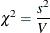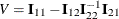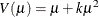Lagrange Multiplier Statistics

When you select the NOINT or NOSCALE option, restrictions are placed on the intercept or scale parameters. Lagrange multiplier, or score, statistics are computed in these cases. These statistics assess the validity of the restrictions, and they are computed aswhere s is the component of the score vector evaluated at the restricted maximum corresponding to the restricted parameter and. The matrixis the information matrix, 1 refers to the restricted parameter, and 2 refers to the rest of the parameters.

Under regularity conditions, this statistic has an asymptotic chi-square distribution with one degree of freedom, and p-values are computed based on this limiting distribution.

If you set k = 0 in a negative binomial model, s is the score statistic of Cameron and Trivedi (1998) for testing for overdispersion in a Poisson model against alternatives of the form.

See Rao (1973, p. 417) for more details.